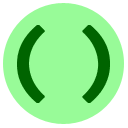# 8: Remix

This lesson consists of several exercises that combine the different skills you learned in the previous lessons.Write a program that takes a single input line of the form `«number1»+«number2»`, where both of these represent positive integers, and outputs the sum of the two numbers. For example on input `5+12` the output should be `17`. Hint 1Hint 2
You may enter input for the program in the box below.Coding Exercise: Substring Counting
As mentioned in lesson 7A, a substring is any consecutive sequence of characters inside another string. The same substring may occur several times inside the same string: for example "assesses" has the substring "sses" 2 times, and "trans-Panamanian banana" has the substring "an" 6 times. Write a program that takes two lines of input, we call the first `needle` and the second `haystack`. Print the number of times that `needle` occurs as a substring of `haystack`. Hint
You may enter input for the program in the box below.

In the following question, once you get it correct we'll show you a graphical representation of the output using `*` graphics.Coding Exercise: Watch the Pendulum
In physics, for a pendulum with length L and initial angle A, its horizontal displacement X(T) at time T is given by the formula

X(T) = L × cos(A × cos(T × √9.8/L)) - L × cos(A)

Write a program which takes two lines of input; the first line is L and the second line is A. The output should be ten lines, giving the values of X(0), X(1), X(2), ..., X(9). For example, if the first line of input is `53.1` and the second line of input is `0.8`, then the first line of output is `0.0` and the second line of output is 53.1*cos(0.8*cos(1*√9.8/53.1)) - 53.1*cos(0.8) ~ `2.6689`.

You may enter input for the program in the box below.Coding Exercise: Centering Text
For this program, the first line of input is an integer `width`. Then, there are some lines of text; the line `"END"` indicates the end of the text. For each line of text, you need to print out a centered version of it, by adding periods `..` to the left and right, so that the total length of each line of text is `width`. (All input lines will have length at most `width`.) Centering means that the number of periods added to the left and added to the right should be equal if possible; if needed we allow one more period on the left than the right. For example, for input

`13Textinthemiddle!END`
the correct output would be

`.....Text..........in..........the........middle!...`
Hint
You may enter input for the program in the box below.Coding Exercise: Ending Time
This program takes two lines of input. The first line is a "starting time" expressed in a 24-hour clock with leading zeroes, like `08:30` or `14:07`. The second line is a duration D in minutes. Print out what time it will be D minutes after the starting time. For example, for input

`12:3047`
the correct output would be `13:17`. All times should be formatted as numbers between 00:00 and 23:59, but the time period may go over midnight. For example, on input

`23:5913`
the correct output is `00:12`. Hints: #1 #2
You may enter input for the program in the box below.Coding Exercise: Character Map
Several lessons ago we showed you the following diagram:

```chr:      !   "   #   \$   %   &   '   (   )   *   +   ,   -   .   /
asc: 32  33  34  35  36  37  38  39  40  41  42  43  44  45  46  47
chr:  0   1   2   3   4   5   6   7   8   9   :   ;   <   =   >   ?
asc: 48  49  50  51  52  53  54  55  56  57  58  59  60  61  62  63
chr:  @   A   B   C   D   E   F   G   H   I   J   K   L   M   N   O
asc: 64  65  66  67  68  69  70  71  72  73  74  75  76  77  78  79
chr:  P   Q   R   S   T   U   V   W   X   Y   Z   [   \   ]   ^   _
asc: 80  81  82  83  84  85  86  87  88  89  90  91  92  93  94  95
chr:  `   a   b   c   d   e   f   g   h   i   j   k   l   m   n   o
asc: 96  97  98  99  100 101 102 103 104 105 106 107 108 109 110 111
chr:  p   q   r   s   t   u   v   w   x   y   z   {   |   }   ~
asc: 112 113 114 115 116 117 118 119 120 121 122 123 124 125 126 127 ```
Write a program which prints out this table. (Character 127 is invisible but should be printed out like all the other characters anyway. Extra/missing space characters at the end of each line don't matter, the grader will ignore them.)
You may enter input for the program in the box below.